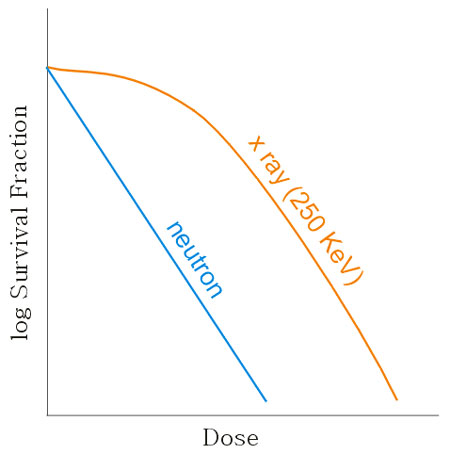##### Radiobiology > Biologic Interactions > Cel Survival Curve > Introduction

Cell Survival CurveThe cell survival curve is the plot of log surviving fraction versus dose...

How is a cell survival curve established?

• Visualize a set of Petri plates each having a known number of clonogenic cells in an environment that will support cell proliferation. A control dish receives no radiation. Successive plates receive increasing doses of X-rays. The plates are incubated until each surviving cell (clonagen) has produced a macroscopic colony. The colonies on each plate are counted.
• For each dose, a surviving fraction, S, may be calculated as: the number of colonies receiving the dose divided by the number of colonies on the control plate.Cell survival curves for neutrons and x-rays. In the above example, the biologic effect is surviving fraction of cell colonies. X-rays of 250 KeV are the usual standard for comparison – so, RBE may be read as “the dose multiplier for 250 KeV x-rays to give identical biologic effect.”  In most situations, the cell survival curve can be modeled as a linear-quadratic relationship.

Note that this curve visually demonstrates Relative Biologic Effectiveness (RBE) – a comparator of different particles or photons – which is the ratio of doses giving identical biologic effect.

For low doses, the survival curve of x-rays has a shoulder: reflecting an ability of cells to repair damage at low dose x-ray exposures (i.e., sub-lethal damage).

By contrast, the neutron curve does not demonstrate such a shoulder – thus, RBE is greatest at these lower doses and becomes constant at higher doses where both dose-response curves are log-linear.